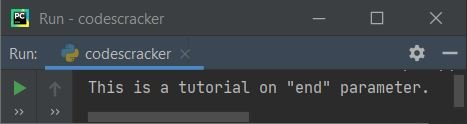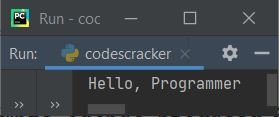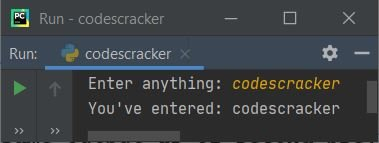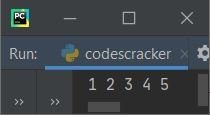# Python end (end=) Parameter in print()

This article is created to cover an interesting topic in Python: the "end" parameter of print(). Many Python programs use the end (end=). As a result, we must comprehend it.

The end parameter is used to change the default behavior of the print() statement in Python. That is, since print() automatically inserts a newline after each execution. As a result, using the end can cause this to change. The end parameter is sometimes used to customize the output console.

## Benefits of the end parameter in Python

We can use "end" to skip the insertion of an automatic newline. But we can also use it to insert one, two, or multiple newlines using a single "print()." The examples given below show the use of "end" in every aspect.

## Python end Parameter Syntax

Because "end" is a parameter of the print() statement or function, its syntax is not unique. That is, the syntax to use "end" looks like this:

`print(end="")`

Inside the double quote, you can insert whatever you want, according to your needs, such as a space, no space, comma, newline ('\n'), etc.

## Python end parameter example

This section includes a few examples that will help you fully understand the topic. Let's start with a very basic one, given below.

### end parameter without a space or newline

This program employs "end," which results in no space and no newline.

```print("Py", end="")
print("thon")```

The above program produces the output shown in the snapshot given below:### parameter ending with a space and no newline

The program given below shows how to add a single or multiple spaces with no newline in a Python program:

```print("This is a tutorial on", end=" ")
print("\"end\" parameter.")```

The snapshot given below shows the sample output produced by the above program:### end parameter with a comma, a space, and no newline

This is the third example of a program with an "end" parameter. This program demonstrates how to use "end" to add multiple items:

```print("Hello", end=", ")
print("Programmer")```

This program produces:Note: Basically, whatever you provide inside the double quote of end="". You will get the respective output.

### end parameter with one, two, or multiple newlines

Since the print() statement, by default, always inserts and prints a single newline, but using "end" as its parameter, we can change this to print the required number of newlines, as shown in the program given below:

```print("Hey", end="!\n\n")
print("What's Up", end="\n")
print("Great to see you learning Python here.", end="\n\n\n\n")
print("Thank You!")```

Now the output produced by this Python program is exactly the same as shown in the snapshot given below:You can also put some message or content for print() inside its "end" parameter, like shown in the program given below:

```print("H", end="ey!\n\n")
print("What", end="'s Up\n")
print("Great to see you ", end="learning Python here.\n\n\n\n")
print("Thank", end=" You!\n")```

This program produces the same output as the previous program's output.

### Putting everything from print() inside the end parameter

You can also put all the things inside the "end," like shown in the program given below:

```print(end="Hey!\n\n")
print(end="What's Up\n")
print(end="Great to see you learning Python here.\n\n\n\n")
print(end="Thank You!\n")```

Again, this program produces the same output as the second previous program.

Note: Basically, the "end" parameter of print() forces it not to automatically insert a newline.

### end parameter provides interactive output for user input

This type of program, where the "end" parameter is used when asking the user to enter some inputs, may be seen a lot of times to receive the input on the same line, where the asking message is written as shown in the program given below:

```print("Enter anything: ", end="")
val = input()
print("You've entered:", val)```

Here is its sample run with user input codescracker:### To print list items in a single line, use the end parameter

This is the task, where we almost want to use "end" every time. Because when we've a list of large number of elements, then it takes large number of lines, that looks weird sometime. As a result, we can use "end" to modify it as shown in the program below:

```nums = [1, 2, 3, 4, 5]
for n in nums:
print(n, end=" ")```

This program produces:Python Online Test

« Previous Tutorial Next Tutorial »